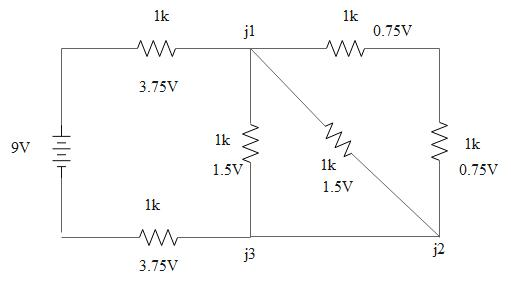The j2 to j3 leg we add a zero resistor

I will break down the circuit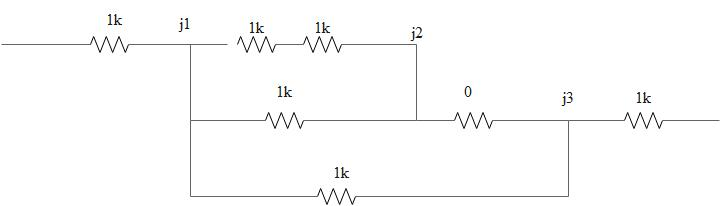We will join the j1 to j2 series resistors

Now combine J1 to J3 resistors in series : 670 + 0 = 670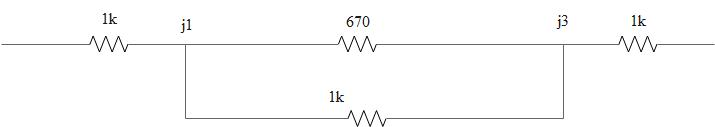Now combine j1 and j3 in parallel.
1/R = 1/670 + 1/1000
R = 401.2

Add what is left in series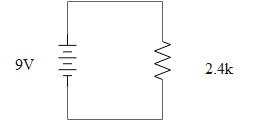now we can get the current of the circuit : I o = 9V / 2400Ω

I o = 3.75mA

We can now figure out the voltage drops

V 1000 = 1kΩ * 3.75mA = 3.75V
V 401 = 401.2Ω * 3.75mA = 1.5V
V 1000 = 1kΩ * 3.75mA = 3.75V

Series resistors have same current but different voltagesj1 to J3 is made up of two resistors 670Ω and 1kΩ.
V 401 = 401.2Ω * 3.75mA = 1.5V

So this voltage goes through both resistors
V 670 = 1.5V and V1000 = 1.5V

I670 = 1.5V / 670Ω = 2.24mA
I1000 = 1.5V / 1000 = 1.5mA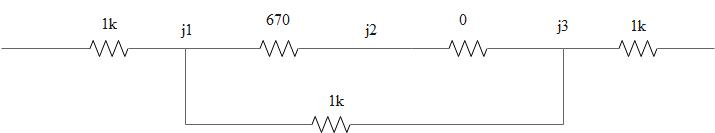Zero resistor has no voltage or current.  It was a place holder.

Resistors in parallel have same voltages but different currents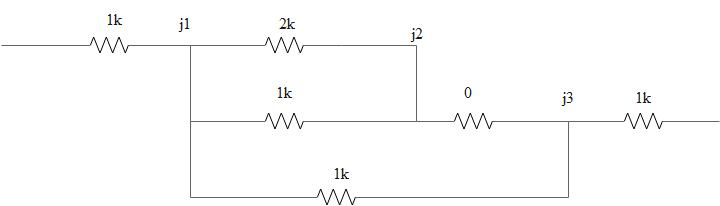The current from j1 to j2

I2000 = 1.5V/2000Ω = 0.75mA
I1000 =1.5V / 1000Ω = 1.5mA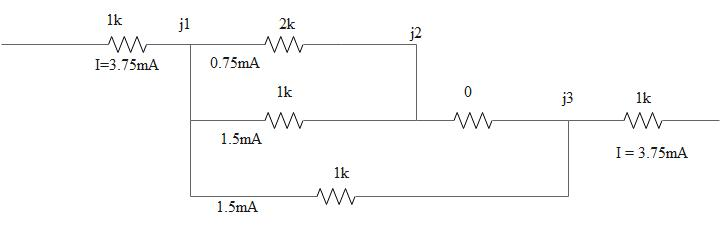Last redraw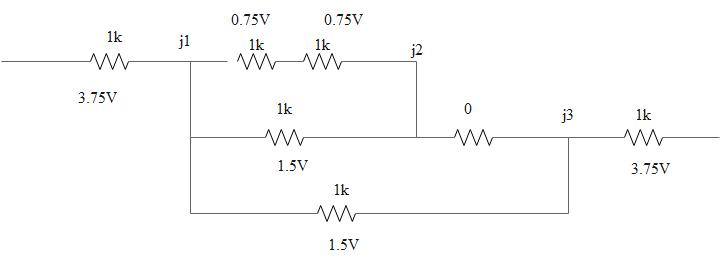Final circuit